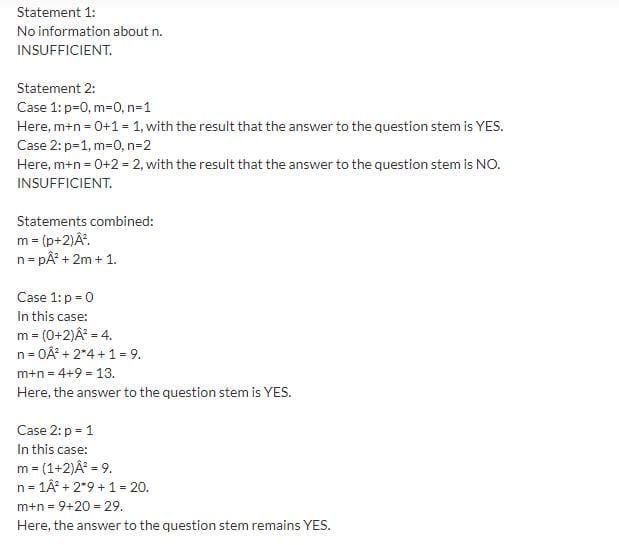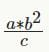# Test: Even And Odds-1

## 25 Questions MCQ Test Quantitative Reasoning for GMAT | Test: Even And Odds-1

Description
Attempt Test: Even And Odds-1 | 25 questions in 50 minutes | Mock test for GMAT preparation | Free important questions MCQ to study Quantitative Reasoning for GMAT for GMAT Exam | Download free PDF with solutions
QUESTION: 1

### Is z even? 1) z/2 is even 2) 3z is even

Solution:

(1) Z = 2 * even
=> z is even
Sufficient

(2) 3z = even
3 is odd
So z is even if z is an integer
But if z = 8/3
Not Sufficient

QUESTION: 2

Solution:
QUESTION: 3

### What is the ratio of number of odd integers to the number of even integers between -10.5 and 10.5?

Solution:

Integers between -10.5 and 10.5 are

-10, -9, -8, -7, -6, -5, -4, -3, -2, -1, 0, 1, 2, 3, 4, 5, 6, 7, 8, 9, 10

Odd integers are -9, -7, -5, -3, -1, 1, 3, 5, 7, 9 (Total 10)

Even integers are -10, -8, -6, -4, -2, 0, 2, 4, 6, 8, 10 (Total 11)

Hence, ratio of number of odd integers to the number of even integers between -10.5 and 10.5 is 10/11

QUESTION: 4

If m is an integer, is m odd?

1) m/2 is not an even integer

2) m – 3 is not an even integer

Solution:
QUESTION: 5

If n is a positive integer, then n (n + 1)(n + 2) is which of the following?

Solution:

The problem is easiest to solve by substituting numbers for n. We’ll try an odd number first and then an even number.

For an odd number, let’s let n = 1: 1(1+1)(1+2) = 1(2)(3) = 6.
We see than choices A and C can’t be the correct choices. Choice A is false because, while the product is even, n is not even. Choice C is false because, while n is odd, the product is even.

For an even number, let’s let n = 2: 2(2+1)(2+2) = 2(3)(4) = 24.

We see than choices B and D can’t be the correct choices. Choice B is false because, while the product is even, n is not odd. Choice D is false because, while the product is divisible by 3, n is even.

Therefore, the only correct answer is choice E. When n is even, the product is indeed divisible by 4.

QUESTION: 6

The product of integers x, y, and z is even. Is z even?

1) x/y = z

2) z = xy

Solution:
QUESTION: 7

The product of the units, tens, and hundreds digits of the positive 3-digit integer x is 42. Is x even?

(1)  x is less than 300. ?

(2)  The tens digit of x is 7. ?

Solution:

Explanation : If the product of the three digits is 42 then the possible set of three digits is 2,3,7 or 6,7,1

Numbers from 2,3,7 are 237,273,327,372,723,732

Numbers from 6,7,1 are 167,176,617,671,716,761

Numbers less than 300 are 237,273,167,176 so x can be both even or odd- Insufficient.

Tens digit is 7

Numbers can be 273,372,671,176.. x can be both even and odd -Insufficient

Taking both the statements together

Number <300 and tens digit is 7 nos are 273,176 both even and odd possible so Insufficient

QUESTION: 8

If m, n, and p are integers, is m+n odd??
(1) m = p2 + 4p + 4
(2) n = p2 + 2m + 1

Solution:So both are required

QUESTION: 9

Is the positive integer p even?

(1) p2 + p is even.

(2) 4p + 2 is even.

Solution:
QUESTION: 10

If p and q are integers and p + q + p is odd, which of the following must be odd?

Solution:

q must be odd, e.g. follwing patterns;

2+1+2 = 5
1+3+1 = 5
1+2+1 = 4
2+4+2 = 8

Hence all combinations with an even q would yield even results, thus q must be odd.

QUESTION: 11

If a , b, and c are integers and ab2 / c is a positive even integer, which of the following must be true?

I. ab is even

II. ab > 0

III. c is even?

Solution:

Given:= even > 0 ab= c* even = even--> either a is even or b or both.

I. ab is even --> according to the above this must be true;

II. ab > 0 --> not necessarily true, bb could be positive as well as negative (for example a=1, c = 1 and b =−2);

III. c is even --> not necessarily true, see above example.

QUESTION: 12

If k and y are integers, and 10k + y is odd, which of the following must be true?

Solution:
QUESTION: 13

Each digit in the two-digit number G is halved to form a new two-digit number H. Which of the following could be the sum of G and H? ?

Solution:
QUESTION: 14

If positive integers x and y are not both odd, which of the following must be even?

Solution:

Positive integers x and y are NOT both odd, means that either both x and y are even or one is even and the other one is odd. In either case xy must be even.

QUESTION: 15

If i and j are integers, is i + j even?

1) i < 10

2) i = j

Solution:
QUESTION: 16

If n is an integer, is n even?

1) n2 – 1 is an odd integer

2) 3n + 4 is an even integer

Solution:
QUESTION: 17

If x and y are both integers, is xy even?

1) x + y is odd

2) x is even

Solution:

Explanation : In order the product of two integers to be even either (or both) of them must be even. So, the question basically asks whether either x or y is even.

(1) x = y + 1. If x is odd then y is even and vise-versa. Sufficient.

(2) x is even

Let x = 2, y= 3

x*y = 2*3 = 6 (Even), Sufficient

QUESTION: 18

If x and y are integers, is xy even?

1) x = y + 1

2) x/y is an even integer

Solution:

If either x or y is zero, then xy=0=even, because zero is an even integer. Zero is nether positive nor negative, but zero is definitely an even number.

An even number is an integer that is "evenly divisible" by 2, i.e., divisible by 2 without a remainder and as zero is evenly divisible by 2 then it must be even (in fact zero is divisible by every integer except zero itself).

QUESTION: 19

Is p2 an odd integer?

1) p is an odd integer

2) √p is an odd integer

Solution:
QUESTION: 20

If x and y are prime integers and x < y, which of the following cannot be true??

Solution:

2X+Y===Even +odd= odd...Always False
(As x <y then x can be smallest prime 2 leaving only odd prime choices for y)

QUESTION: 21

If x and y are positive integers, is the product xy even?

1) 5x - 4y is even

2) 6x + 7y is even

Solution:

1) 4y will always be even. Then we have 5x−even=even ,

For this to be the case, 5x must be even. Since 5 can't be even, then x must be even. Thus the product xy will be even. Sufficient.

2) 6x will always be even. Then we have even+7y=even.

Thus 7y is even, and y is even, and xy is even. Sufficient.

QUESTION: 22

If x and y are integers, is x (y + 1) an even number?

1) x and y are prime numbers.

2) y > 7 ?

Solution:
QUESTION: 23

For all positive integers m, (m) = 3m when m is odd and (m) = (1⁄2) m when m is even, which of the following is equivalent to (9)*(6)?

Solution:

Notice that [ ] is just some function such that "[m]=3m when m is odd and [m]=(1/2)*m when m is even".

So, [m]=3m when m is odd, [m]=(1/2)*m when m is even.
As 9 is odd then  equals to 3*9=27;
As 6 is even then  equals to 1/2*6=3;

So *=27*3=81. Note that numbers in the answer choices are also in boxes, so we have: [m]=81. m could be 27 (in this case as 27 is odd =3*27=81) OR 162 (in this case as 162 is even =162/2=81) --> only  is in the answer choices.

QUESTION: 24

If m and n are integers, is m odd??

1) m + n is odd

2) m + n = n2 + 5

Solution:

(1) n + m is odd

The sum of two integers is odd only if one is odd and another is even, hence m may or may not be odd. Not sufficient.

(2) n + m = n2 + 5

-->  m−5=n2−n

=> m−5=n(n−1)

either n or n−1 is even hence n(n−1)=even

=> n(n−1)=even

=> m−5=m−odd=even

--> m=odd. Sufficient.

QUESTION: 25

For non-negative integers x, y, and z, is xz odd?

1) xz = odd

2) x = 2y

Solution:

(1) The product xz is odd.
=> x, z are odd => xz  odd => SUF

(2) x = 2y
=> x is even => xz even => SUFUse Code STAYHOME200 and get INR 200 additional OFF Use Coupon Code